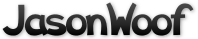Got questions, comments, patches, etc.? Contact Jason Woofenden
1 #include <math.h>
2 #include <stdlib.h>
3 #include <string.h>
4 #include "config.h"
5 #include "common.h"
6 #include "globals.h"
7 #include "sprite.h"
8 #include "rocks.h"
13 // 2 sets of sprites, sorted by position
14 static Sprite **sprites = { NULL, NULL };
16 // which set are we using?
17 static int set = 0;
19 // size of squares into which sprites are sorted.
20 static int grid_size = 0;
22 // screen size in grid squares.
23 static int gw = 0, gh = 0;
25 // lists of free sprites, by type.
26 Sprite *free_sprites[N_TYPES];
29 static void
30 get_shape(Sprite *s)
31 {
32         int x, y;
33         uint16_t *px, transp;
34         uint32_t bits = 0, bit, *p;
36         s->area = 0;
37         if(s->image->format->BytesPerPixel != 2) {
38                 fprintf(stderr, "get_shape(): not a 16-bit image!\n");
39                 exit(1);
40         }
42         s->w = s->image->w; s->h = s->image->h;
43         grid_size = max(grid_size, max(s->w, s->h));
47                 fprintf(stderr, "get_shape(): can't allocate bitmask.\n");
48                 exit(1);
49         }
51         SDL_LockSurface(s->image);
52         px = s->image->pixels;
53         transp = s->image->format->colorkey;
55         for(y=0; y<s->image->h; y++) {
56                 bit = 0;
57                 for(x=0; x<s->image->w; x++) {
58                         if(!bit) { bits = 0; bit = 0x80000000; }
59                         if(*px++ != transp) { bits |= bit; s->area++; }
60                         bit >>= 1;
61                         if(!bit || x == s->image->w - 1) { *(p++) = bits; }
62                 }
63                 px = (uint16_t *) ((uint8_t *) px + s->image->pitch - 2*s->image->w);
64         }
65         SDL_UnlockSurface(s->image);
66 }
69 void
71 {
73         if(s->image) get_shape(s);
74 }
77 static void
79 {
82 }
85 void
86 init_sprites(void)
87 {
90         grid_size = grid_size * 3 / 2;
91         gw = (XSIZE-1 + 2*grid_size) / grid_size;
92         gh = (YSIZE-1 + 2*grid_size) / grid_size;
94         sprites = malloc(2 * gw * gh * sizeof(Sprite *));
95         sprites = (void *)sprites + gw * gh * sizeof(Sprite *);
96         if(!sprites) {
97                 fprintf(stderr, "init_sprites(): can't allocate grid squares.\n");
98                 exit(1);
99         }
100         memset(sprites, 0, 2 * gw * gh * sizeof(Sprite *));
101         set = 0;
102 }
104 static inline Sprite **
105 square(int x, int y, int set)
106 {
107         int b = (x+grid_size)/grid_size + gw*((y+grid_size)/grid_size);
108         return &sprites[set][b];
109 }
111 void
113 {
114         insert_sprite(square(s->x, s->y, set), s);
115 }
117 void
118 reset_sprites(void)
119 {
120         int i;
122         for(i=0; i<gw*gh; i++)
123                 while(sprites[set][i]) {
124                         Sprite *s = remove_sprite(&sprites[set][i]);
125                         insert_sprite(&free_sprites[s->type], s);
126                         s->flags = 0;
127                 }
128 }
130 void
131 move_sprite(Sprite *s)
132 {
133         if(s->flags & MOVE) {
134                 s->x += (s->dx - screendx)*t_frame;
135                 s->y += (s->dy - screendy)*t_frame;
136         }
137 }
139 void
140 sort_sprite(Sprite *s)
141 {
142         // clip it, or sort it into the other set of sprites.
143         if(s->x + s->w < 0 || s->x >= XSIZE
144            || s->y + s->h < 0 || s->y >= YSIZE) {
145                 insert_sprite(&free_sprites[s->type], s);
146                 s->flags = 0;
147         } else insert_sprite(square(s->x, s->y, 1-set), s);
148 }
150 void
151 move_sprites(void)
152 {
153         int sq;
156         // Move all the sprites
157         for(sq=0; sq<gw*gh; sq++) {
161                         move_sprite(s); sort_sprite(s);
162                 }
163         }
164         set = 1-set;  // switch to other set of sprites.
165 }
168 static int
170 {
171         int i, words = (xov-1) >> 5;
172         uint32_t abits;
174         for(i=0; i<words; i++) {
175                 abits = *amask++ << bit;
176                 abits |= *amask >> (32-bit);
177                 if(abits & *bmask++) return true;
178         }
179         abits = *amask << bit;
180         if(abits & *bmask) return true;
182         return false;
183 }
185 static int
186 mask_collide(int xov, int yov, Sprite *a, Sprite *b)
187 {
188         int y;
189         int xoffset = a->w - xov;
190         int word = xoffset >> 5, bit = xoffset & 31;
193         if(yov > 0) {
196         } else {
197                 yov = -yov;
200         }
202         for(y=0; y<yov; y++) {
205         }
207         return 0;
208 }
210 int
211 collide(Sprite *a, Sprite *b)
212 {
213         int dx, dy, xov, yov;
215         if(!COLLIDES(a) || !COLLIDES(b)) return false;
217         if(b->x < a->x) { Sprite *tmp = a; a = b; b = tmp; }
219         dx = b->x - a->x;
220         dy = b->y - a->y;
222         xov = max(min(a->w - dx, b->w), 0);
224         if(dy >= 0) yov = max(min(a->h - dy, b->h), 0);
225         else yov = -max(min(b->h - -dy, a->h), 0);
227         if(xov == 0 || yov == 0) return false;
228         else return mask_collide(xov, yov, a, b);
229 }
231 void
232 collide_with_list(Sprite *s, Sprite *list)
233 {
234         for(; list; list=list->next)
235                 if(collide(s, list)) do_collision(s, list);
236 }
238 void
239 collisions(void)
240 {
241         int i, end = gw*gh;
242         Sprite *s;
243         for(i=0; i<end; i++) {
244                 for(s=sprites[set][i]; s; s=s->next) {
245                         collide_with_list(s, s->next);
246                         if(i+1 < end) collide_with_list(s, sprites[set][i+1]);
247                         if(i+gw < end) collide_with_list(s, sprites[set][i+gw]);
248                         if(i+gw+1 < end) collide_with_list(s, sprites[set][i+gw+1]);
249                 }
250         }
251 }
253 Sprite *
254 hit_in_square(Sprite *r, Sprite *s)
255 {
256         for(; r; r=r->next)
257                 if(collide(r, s)) break;
258         return r;
259 }
261 Sprite *
262 collides(Sprite *s)
263 {
264         int l, r, t, b;
265         Sprite **sq;
266         Sprite *c;
268         l = (s->x + grid_size) / grid_size;
269         r = (s->x + s->w + grid_size) / grid_size;
270         t = (s->y + grid_size) / grid_size;
271         b = (s->y + s->h + grid_size) / grid_size;
272         sq = &sprites[set][l + t*gw];
274         if((c = hit_in_square(*sq, s))) return c;
275         if(l > 0 && (c = hit_in_square(*(sq-1), s))) return c;
276         if(t > 0 && (c = hit_in_square(*(sq-gw), s))) return c;
277         if(l > 0 && t > 0 && (c = hit_in_square(*(sq-1-gw), s))) return c;
279         if(r > l) {
280                 if((c = hit_in_square(*(sq+1), s))) return c;
281                 if(t > 0 && hit_in_square(*(sq+1-gw), s)) return c;
282         }
283         if(b > t) {
284                 if((c = hit_in_square(*(sq+gw), s))) return c;
285                 if(l > 0 && (c = hit_in_square(*(sq-1+gw), s))) return c;
286         }
287         if(r > l && b > t && (c = hit_in_square(*(sq+1+gw), s))) return c;
288         return NULL;
289 }
291 int
292 pixel_collide(Sprite *s, int x, int y)
293 {
296         if(!COLLIDES(s)) return false;
298         if(x < s->x || y < s->y || x >= s->x + s->w || y >= s->y + s->h) return 0;
300         x -= s->x; y -= s->y;
301         pmask = 0x80000000 >> (x&0x1f);
303 }
305 Sprite *
306 pixel_hit_in_square(Sprite *r, float x, float y)
307 {
308         for(; r; r=r->next) {
309                 if(COLLIDES(r) && pixel_collide(r, x, y)) return r;
310         }
311         return 0;
312 }
314 Sprite *
315 pixel_collides(float x, float y)
316 {
317         int l, t;
318         Sprite **sq;
319         Sprite *ret;
321         l = (x + grid_size) / grid_size; t = (y + grid_size) / grid_size;
322         sq = &sprites[set][l + t*gw];
323         if((ret = pixel_hit_in_square(*sq, x, y))) return ret;
324         if(l > 0 && (ret = pixel_hit_in_square(*(sq-1), x, y))) return ret;
325         if(t > 0 && (ret = pixel_hit_in_square(*(sq-gw), x, y))) return ret;
326         if(l > 0 && t > 0 && (ret = pixel_hit_in_square(*(sq-1-gw), x, y))) return ret;
327         return 0;
328 }
331 float
332 sprite_mass(Sprite *s)
333 {
334         if(s->type == SHIP) return s->area;
335         else if(s->type == ROCK) return 3 * s->area;
336         else return 0;
337 }
339 /*
340  * BOUNCE THEORY
341  *
342  * ******************  In 1 Dimension  *****************
343  *
344  * For now we will imagine bouncing A and B off each other in 1 dimension (along
345  * a line). We can safely save the other dimension for later.
346  *
347  * A and B are the same weight, and are both traveling 1m/sec, to collide right
348  * at the origin. With perfect bounciness, their full momentum is reversed.
349  *
350  * If we cut the weight of A down by half, then the center of our colision will
351  * drift towards A (the speeds of A and B are not simply reversed as in our last
352  * example.) However, there is always a place between A and B on the line (I'll
353  * call it x) such that the speeds of A and B relative to x, are simply
354  * reversed. Thus we can find the new speed for A like so:
355  *
356  *     new A = x -(A - x)
357  *
358  *     new B = x -(B - x)
359  *
360  * or, simply:
361  *
362  *     new A = 2x - A
363  *
364  *     new B = 2x - B
365  *
366  *
367  * this point x is the sort of center of momentum. If, instead of bouncing, A
368  * and B just globbed together, x would be center of the new glob.
369  *
370  * x is the point where there's an equal amount of force coming in from both
371  * sides. ie the weighted average of the speeds of A and B.
372  *
373  * average force = (A force + B force) / total mass
374  *
375  * x.speed = (a.speed * a.mass + b.speed * b.mass) / (a.mass + b.mas)
376  *
377  * then we apply the formula above for calculating the new A and B.
378  *
379  *
380  *
381  *
382  * ******************  In 2 Dimensions  *****************
383  *
384  * OK, that's how we do it in 1D. Now we need to deal with 2D.
385  *
386  * Imagine (or draw) the two balls just as they are bouncing off each other.
387  * Imagine drawing a line through the centers of the balls. The balls are
388  * exerting force on each other only along this axis. So if we rotate
389  * everything, we can do our earlier 1D math along this line.
390  *
391  * It doesn't matter what direction the balls are going in, they only exert
392  * force on each other along this line. What we will do is to compute the part
393  * of the balls' momentum that is going along this line, and bounce it according
394  * to our math above. The other part is unaffected by the bounce, and we can
395  * just leave it alone.
396  *
397  * To get this component of the balls' momentum, we can use the dot product.
398  *
399  *     dot(U, V) = length(U) * length(V) * cos(angle between U and V)
400  *
401  * If U is a length 1 vector, then dot(U, V) is the length of the component of V
402  * in the direction of U.  So the components of V are:
403  *
404  *     U * dot(U, V)      parallel to U
405  *
406  *     V - U * dot(U, V)  perpendicular to U
407  *
408  * To do the actual bounce, we compute the unit vector between the center of the
409  * two balls, compute the components of the balls' speeds along this vector (A
410  * and B), and then bounce them according to the math above:
411  *
412  *     new A = 2x - A
413  *
414  *     new B = 2x - B
415  *
416  * But we rewrite it in relative terms:
417  *
418  *     new A = A + 2(x-A)
419  *
420  *     new B = B + 2(x-B)
421  */
423 void
424 bounce(Sprite *a, Sprite *b)
425 {
426         float x, y, n;  // (x, y) is unit vector from a to b.
427         float va, vb;   // va, vb are balls' speeds along (x, y)
428         float ma, mb;   // ma, mb are the balls' masses.
429         float vc;       // vc is the "center of momentum"
431         // (x, y) is unit vector pointing from A's center to B's center.
432         x = (b->x + b->w / 2) - (a->x + a->w / 2);
433         y = (b->y + b->h / 2) - (a->y + a->h / 2);
434         n = sqrt(x*x + y*y); x /= n; y /= n;
436         // velocities along (x, y)
437         va = x*a->dx + y*a->dy;
438         vb = x*b->dx + y*b->dy;
439         if(vb-va > 0) return;  // don't bounce if we're already moving away.
441         // get masses and compute "center" speed
442         ma = sprite_mass(a); mb = sprite_mass(b);
443         vc = (va*ma + vb*mb) / (ma+mb);
445         // bounce off the center speed.
446         a->dx += 2*x*(vc-va); a->dy += 2*y*(vc-va);
447         b->dx += 2*x*(vc-vb); b->dy += 2*y*(vc-vb);
448 }